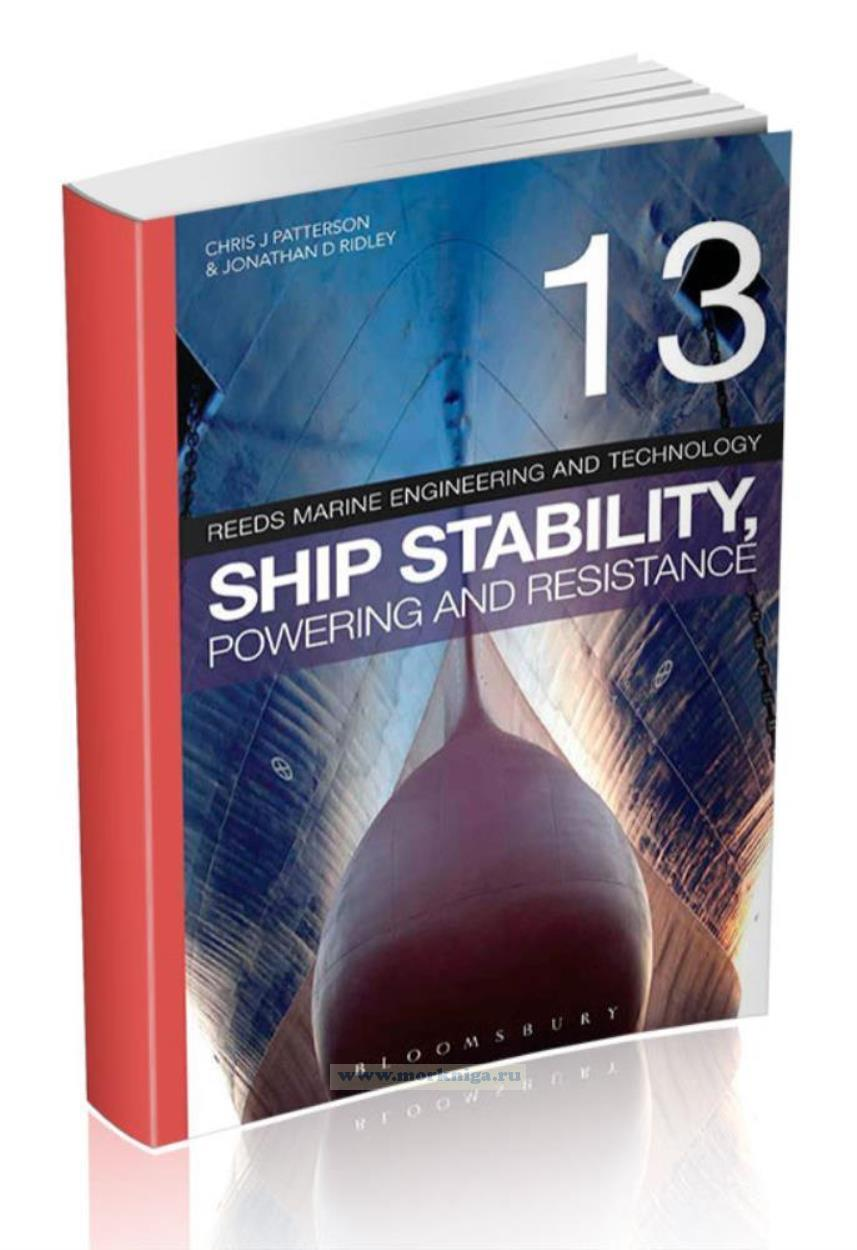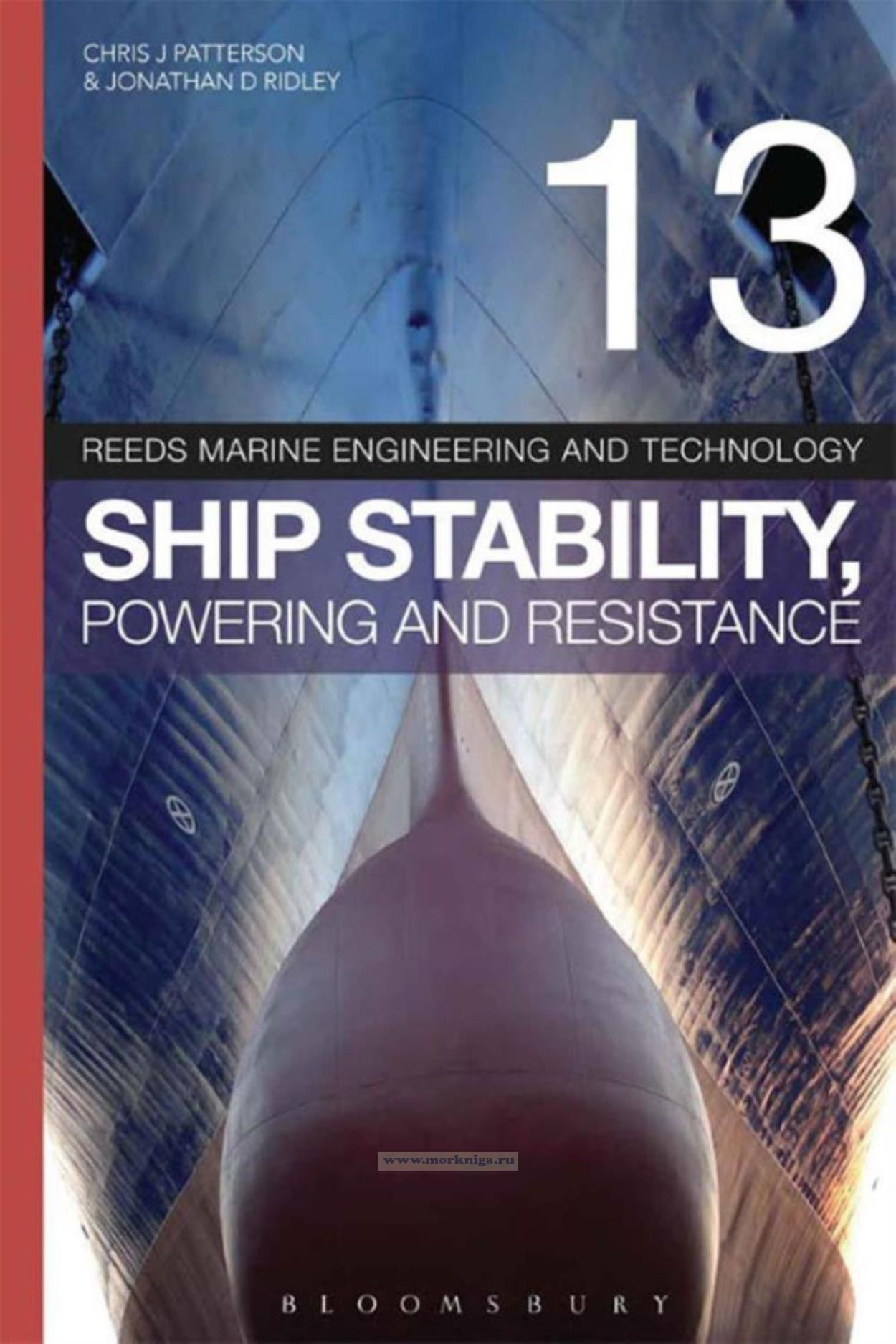звоните нам Пн-Пт с 9 до 19,
Сб с 10 до 16
Каталог

# Ship stability, powering and resistance/Стабильность, мощность и сопротивление корабля

••Артикул: 00-01027946
в желания В наличии
Автор: Chris J Patterson, Jonathan D Ridley
Издательство: Adlard Coles Nautical (все книги издательства)
ISBN: 978-1-4081-7612-2
Год: 2014
Переплет: Мягкая обложка
Страниц: 785
3600 v
-
+
С этим товаром покупают
Скачать/полистать/читать on-line

### Читать on-line книгу "Ship stability, powering and resistance/Стабильность, мощность и сопротивление корабля" Chris J Patterson, Jonathan D Ridley на полный экранИздание на английском языке
These notes are written with the intention of helping develop an understanding of stability and propulsion for students of nautical science based degree courses, Merchant Navy Officers undertaking their Officer of the Watch or Master/Chief Mate Stability and Structures examinations, and Marine Engineers' Naval Architecture examinations with the UK Maritime and Coastguard Agency (MCA). Some topic areas are required for some examinations but not others. For each topic area, the titles are marked (OOW), (MCM) or (ENG) indicating the level of the topic.
For each section, the learning aims and objectives are listed at the start of the section, and again at the end of the section as a checklist. You should ensure that you can tick off all of the relevant boxes before the end of the course. These lists are for guidance only - they are not an exhaustive list of all skills, abilities and knowledge required.
Questions within the notesare designed to test knowledge up to that point in the notes, and outline solutions to all of these questions are given in Chapter 14.Try and attempt the questions before looking at the solutions. Questions suitable for the Officer of the Watch level course syllabus are marked (OOW) alongside the question number, while questions suitable for the Master/Chief Mate level course are marked (MCM). Questions suitable for the Marine Engineers' courses are marked (ENG).
Background mathematical proofs and assumptions are covered in the appendices of these notes, so that you can develop an understanding of the underlying physics. These are indicated by arrow symbols.
The most important formulae, needed to solve problems, are marked in bold and are highlighted in boxes.

Contents
Acknowledgements
Nomenclature
Introduction
1 Flotation and buoyancy
2 Small angle or initial metacentric stability will my ship float upright?
3 Large angle stability-just how far can she roll?
4 Longitudinal stability - fore and aft balance
6 Bilging and damaged stability
7 Calculating hull shear force and bending moment
8 Calculating hydrostatics using Simpson's rule
9 Ship resistance
10 Ship propulsion
11 Screw propellers
12 Propeller alternatives for high-speed craft
13 Rudders
14 Solutions to questions
Appendix 1 MV reed - sample stability data booklet
Appendix 2 Derivation of Archimedes' principle for box shaped vessels
Appendix 3 Derivation of Archimedes' principle for semicircular section vessels
Appendix 4 Linear interpolation using the equation of a straight line
Appendix 5 The derivation of the TPC formula
Appendix 6 The derivation of the fresh water allowance formula
Appendix 7 The derivation of the change in kg formula
Appendix 8 The derivation of the formulae giving the transverse inertia of a rectangular waterplane and a ship shape waterplane measured through the centreline
Appendix 9 The derivation of the relationship between transverse inertia, bm and underwater volume for a box shaped vessel
Appendix 10 The derivation of the relationship between transverse inertia, BM and underwater volume for a ship
Appendix 11 Maximum angles of list on passenger vessels due to passenger crowding
Appendix 12 The derivation of the formula giving the angle of list for a neutrally stable vessel
Appendix 13 The derivation of the formulae describing the effect of free surfaces on metacentric height
Appendix 14 Passenger vessel heel in a turn
Appendix 15 Derivation of the increase in draught when heeling formula
Appendix 16 The mathematical proof of the determination of GM from the GZ curve
Appendix 17 The derivation of the angle of heel in a turn formula
Appendix 18 The mathematical proof of Simpson's rule
Appendix 19 Alternative criteria for large angle stability (timber deck cargo)
Appendix 20 The direct calculation of the area under a generic GZ curve
Appendix 21 The derivation of the wall-sided formula for approximating the righting lever
Appendix 22 The derivation of the formula giving the effective metacentric height at an angle of loll
Appendix 23 The derivation of the formula giving the longitudinal inertia of a rectangle
Appendix 24 The derivation of the formula giving the longitudinal BM for a box shaped vessel
Appendix 25 The derivation of the moment to change trim by 1 cm formula
Appendix 26 The derivation of the trim equation
Appendix 27 The derivation of the formula linking cargo density, stowage factor and compartment permeability
Appendix 28 Derivation of the formula giving the transverse inertia of a rectangular waterplane measured from the long edge
Appendix 29 Derivation of the parallel axes theorem (huygens-steiner theorem)
Appendix 30 Direct calculation of shear force and bending moment
Appendix 31 Properties of water
Works cited
Index

#### Здесь Вы можете оставить свой отзыв

Чтобы оставить отзыв на товар Вам необходимо войти или зарегистрироваться